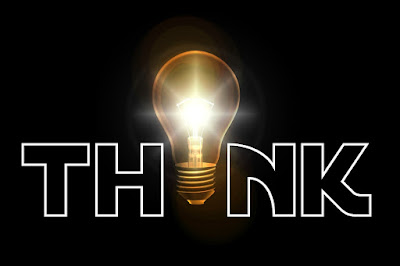## Monday, 11 June 2018

### Control System Multiple Choice Questions

Electronics and communication Multiple Choice Questions

1. Poles are the complex frequencies of a transfer function where the response becomes?
a. infinite
b. zero
c. oscillatory
d. decaying

2. Zeros are the complex frequencies of a transfer function where the response becomes?
a. infinite
b. zero
c. oscillatory
d. decaying

3. Which of the following is an open loop system?
a. ward Leonard control
b. Amplidyne
c. Armature controlled d.c. motor
d. Field controlled d.c. motor

4. A driver maintains the speed of a car at 50 Km per hour. Here the feedback element is?
a. steering wheel
b. needle of the speedometer
c. eyes
d. all of these

5. Transient response of a system is basically because of?
a. coupling
b. forces
c. friction
d. stored energy

6. In a critically damped system, the damping factor of the system is?
a. zero
b. unity
c. less than unity
d. greater than unity

7. With feedback, the transient response of the system as compared to that without feedback?
a. decays slowly
b. decays more quickly
c. rises at a faster rate
d. remain unaltered

8. By increasing the gain k of the system, the steady state error of the system?
a. decreases
b. increases
c. remain unchanged
d. may increase or decreases

9. Integral error compensation is also called?
a. proportional minus integral compensation
b. proportional plus integral compensation
c. proportional plus derivative compensation
d. proportional minus derivative compensation

10. For 2% tolerance band, the settling time the second order linear system is? Where t is the time constant.
a. t/2
b. t
c. 2t
d.  4t

1. a
2. b
3. d
4. b
5. d
6. b
7. b
8. a
9. b
10. d

Share you comments. #gate #psu #eto